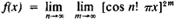# Baire Classification

The following article is from The Great Soviet Encyclopedia (1979). It might be outdated or ideologically biased.

## Baire Classification

(mathematics), a classification of discontinuous functions. The first class in the Baire classification includes any discontinuous function that can be represented as the limit of a sequence of continuous functions that converges at all points (functions of the zero class). This class was studied in detail by the French mathematician R. Baire in 1899; it includes, for example, all functions with a finite number of points of discontinuity. Any discontinuous function that does not belong in the first class but that can be represented as the limit of a convergent sequence of functions of the first class belongs in the second class. An example is the Dirichlet functionequal to 0 for all irrational x and 1 for all rational x. Functions of the third, fourth, and subsequent classes are defined analogously, and the enumeration of the classes can be extended to transfinite numbers rather than being limited to natural (finite) numbers. H. Lebesgue (1905) proved the existence of a function of any class, as well as the existence of a function not belonging to the Baire classification. The theory of functions in the Baire classification (B functions) is closely related to the theory of B -measurable sets (B sets). The B sets were introduced by E. Borel. N. N. Luzin and his students have devoted detailed studies to them.

### REFERENCE

Baire, R. Teoriia razryvnykh funktsii. Moscow-Leningrad, 1932. (Translated from French.)
The Great Soviet Encyclopedia, 3rd Edition (1970-1979). © 2010 The Gale Group, Inc. All rights reserved.
Site: Follow: Share:
Open / Close